## Section38.6Motion in a Magnetic Field

In this section we will study motion of a charged particle in an environment where only magnetic field is present. To further simplify the situation, we will study the motion only in an environement of constant magnetic field.

We will find below that if a particle of charge $Q$ enters a region of constant uniform magnetic field $\vec B$ with velocity $\vec v$ perpendicular to the magnetic field, then, its motion is a circular motion with Newton's law taking the following form.

\begin{equation*} m\dfrac{v^2}{R} = Q v B. \end{equation*}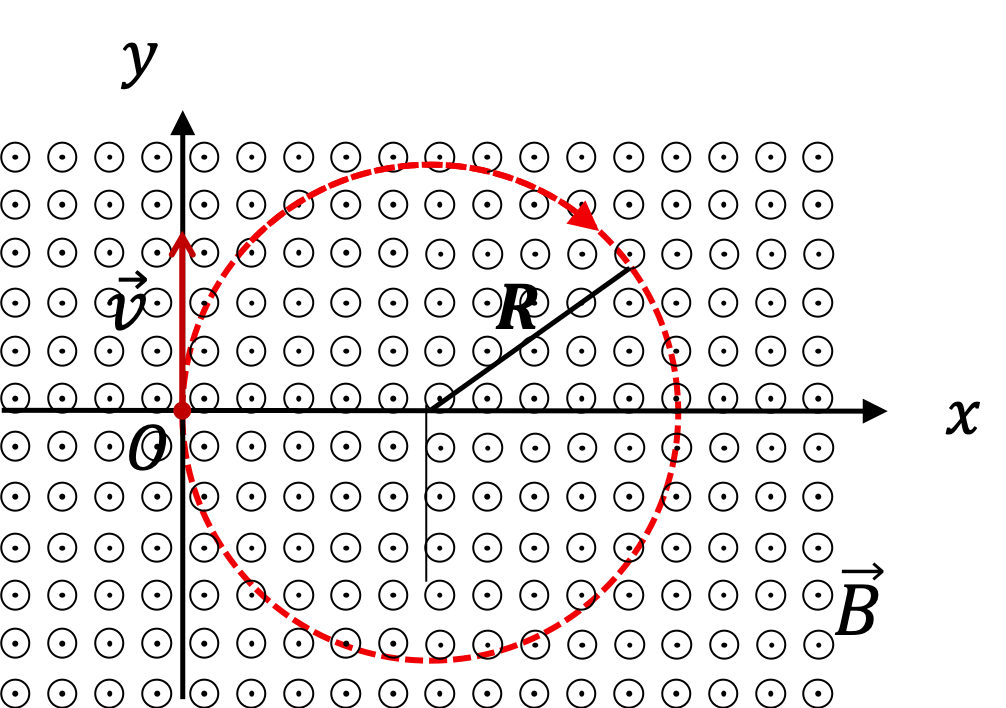Figure 38.6.1. Circular motion of a positive charge in a uniform magnetic field.

This also says that, even though momentum changes as reflected in the chnaging direction of motion, the magnitude of momentum is constant and is given by

\begin{equation*} m v = Q R B. \end{equation*}

Note that the angular speed $\Omega$ in the circular motion is

\begin{equation*} \Omega = \dfrac{v}{R} = \dfrac{QB}{m}. \end{equation*}

Even when the motion may not complete a circle, we can imagine a period of a particle moving continuously in a circle with this angular speed. The period of such an imagined motion will be the time to complete the motion once around the circle, i.e, time for $2\pi$ radians of angle.

\begin{equation*} T = \dfrac{2\pi}{\Omega}. \end{equation*}

the inverse of this period is called cyclotron frequency, $f_c\text{.}$

\begin{equation*} f_c = \dfrac{\Omega}{2\pi}. \end{equation*}

The formulas above are for particles that enter a magnetic field region with velocity perpendicular to the magnetic field. What happens if velocity is not perpendicular to the magnetic field? Suppose magnetic field is along $z$ axis and particle's velocity makes an angle $\theta$ with the $z$ axis. You will find that the particle moves in a helical path around the $z$ axis, as shown in Figure 38.6.2 below, with the pitch $\lambda$ given by

\begin{equation*} \lambda = \dfrac{ 2\pi v\cos\, \theta }{\Omega}, \end{equation*}

and the radius $R$ of the loops in the helix is

\begin{equation*} R = \dfrac{1}{\Omega}v\sin\,\theta. \end{equation*}

### Subsection38.6.1(Calculus) Equation of Motion of a Particle in Uniform Magnetic Field

Consider a particle of mass $m$ and charge Q moving in a uniform magnetic field of magnitude $B_0\text{,}$ which is pointed towards positive $z$ axis. We assume only magnetic forces on the particle are relevant. Then, equation of motion of the particle,

\begin{equation*} m\dfrac{d\vec v}{dt} = Q\vec v \times \vec B, \end{equation*}

takes the following component form.

\begin{align*} \dfrac{dv_x}{dt} \amp = \dfrac{QB_0}{m}\,v_y, \\ \dfrac{dv_y}{dt} \amp = -\dfrac{QB_0}{m}\,v_x, \\ \frac{dv_z}{dt} \amp = 0. \end{align*}

Denote $Q B_0/m$ by symbol $\Omega$ to simplify writing.

\begin{equation*} \Omega = \dfrac{QB_0}{m}. \end{equation*}

Therefore, the equations of motion are

\begin{align} \dfrac{dv_x}{dt} \amp = \Omega\,v_y, \tag{38.6.1}\\ \dfrac{dv_y}{dt} \amp = -\Omega\,v_x, \tag{38.6.2}\\ \frac{dv_z}{dt} \amp = 0. \tag{38.6.3} \end{align}

We wish to solve this equation for given initial velocity, $\vec v_0 = \left( v_{0x},\ v_{0y}\ v_{0z}\right)\text{.}$ First notice that we can get separate equations for v_x and v_y.

\begin{align} \dfrac{d^2v_x}{dt^2} \amp = -\Omega^2\,v_x, \tag{38.6.4}\\ \dfrac{d^2v_y}{dt^2} \amp = -\Omega^2\,v_y, \tag{38.6.5}\\ \frac{dv_z}{dt} \amp = 0. \tag{38.6.6} \end{align}

The solutions are

\begin{align*} \amp v_x(t) = A \cos(\Omega t) + B \sin(\Omega t), \\ \amp v_y(t) = C \cos(\Omega t) + D \sin(\Omega t), \\ \amp v_z(t) = E, \end{align*}

where $A,\ B,\ C,\ D,\ E$ are constants. By using the initial veocity condition, we get

\begin{equation*} E = v_{0z}, \end{equation*}

Note$A,\ B,\ C,\ D$ are not all independent since derivative of $v_x$ is proportional to $v_y\text{.}$ Therefore, we must have

\begin{equation*} -\Omega A \sin(\Omega t) + \Omega B \cos(\Omega t) =\Omega C \cos(\Omega t) + \Omega D \sin(\Omega t). \end{equation*}

Therefore,

\begin{equation*} -A = D,\ \ B = C. \end{equation*}

Therefore, we write $v_x$ and $v_y$ with only two constants.

\begin{align*} \amp v_x(t) = A \cos(\Omega t) + B \sin(\Omega t), \\ \amp v_y(t) = B \cos(\Omega t) - A \sin(\Omega t), \end{align*}

Now, we use initial conditions on these components.

\begin{align*} \amp v_{0x} = A, \\ \amp v_{0y} = B, \end{align*}

Finally, we have velocity at arbitray time

\begin{align*} \amp v_x(t) = v_{0x} \cos(\Omega t) + v_{0y} \sin(\Omega t), \\ \amp v_y(t) = v_{0y} \cos(\Omega t) - v_{0x} \sin(\Omega t), \\ \amp v_z(t) = v_{0z}. \end{align*}

From these we can obtain position of the particle at arbitrary instant by simply integrating. Let position at initial instant be $\vec r_0 = (0,\ 0,\ 0)\text{.}$

\begin{align*} \amp x(t) = \dfrac{v_{0x}}{\Omega} \sin(\Omega t) + \dfrac{v_{0y}}{\Omega} \left(1- \cos(\Omega t) \right), \\ \amp y(t) = \dfrac{v_{0y}}{\Omega} \sin(\Omega t) - \dfrac{v_{0x}}{\Omega} \left( 1 - \cos(\Omega t) \right), \\ \amp z(t) = v_{0z} t. \end{align*}

The trajectory in the $(x,y)$plane, i.e., plane perpendicular the magnetic field, is an equation of a circle with the following center and radius.

\begin{align*} \amp \text{center at: } \left( \dfrac{v_{0y}}{\Omega},\ - \dfrac{v_{0x}}{\Omega} \right)\\ \amp \text{radius: } R = \dfrac{1}{\Omega}\sqrt{ v_{0x}^2 + v_{0y}^2}. \end{align*}

The $z$ coordinate moves linearly in time. As a result, the motion is a helical motion whose axis is parallel to the $z$ axis.

The circular motion perpendicular to the axis of the helix completes one cycle in the time period given by angular frequency $\Omega\text{.}$ Suppose this time is $t_\text{pitch}\text{.}$ Then,

\begin{equation*} t_\text{pitch}= \dfrac{2\pi}{\Omega}. \end{equation*}

During this time, the particle will move a distance $v_{0z}t_\text{pitch}$ along the axis. Therefore, the pitch, $\lambda\text{,}$ of the helical path will be

\begin{equation*} \lambda = v_{0z}\, t_\text{pitch} = \dfrac{2\pi v_{0z}}{\Omega}. \end{equation*}

A particle of charge $+1\;e$ enters a region of uniform magnetic field of magnitude $2\text{ T}$ with a velocity of $9.6 \times 10^5\text{ m/s}$ perpendicular to the magnetic field. It is observed that the particle makes an arc of radius $5\text{ mm}$ before coming out of the region. Find the mass of the particle.

Hint

Use $mv = QRB$ formula.

$1.67\times 10^{-27}\ \text{kg}\text{.}$

Solution

The problem states that the particle makes an arc, which is part of a circle. Therefore, we can claim that the particle describes a circular path when in the region of constant magnetic field and use the formula for $Q/m$ obtained above to write mass in terms of the given quantities.

\begin{equation*} \frac{Q}{m} = \frac{v}{RB_0}\ \ \Longrightarrow \ \ m = \frac{QRB_0}{v} \end{equation*}

Now, we put numbers in to obtain

\begin{align*} m \amp = \frac{1.6\times 10^{-19} \text{C} \times 0.005\ \text{m}\times 2\ \text{T}}{9.6\times 10^{5} \text{m/s}}\\ \amp = 1.67\times 10^{-27}\ \text{kg}. \end{align*}

The particle with charge $+1\ e$ and mass $1.67\times 10^{-27}\ \text{kg}$ is proton.

Consider a region of space where electric field $\vec E$ and magnetic field $\vec B$ are perpendicular to each other as shown in figure below. The perpendicular electric and magnetic fields are called crossed fields.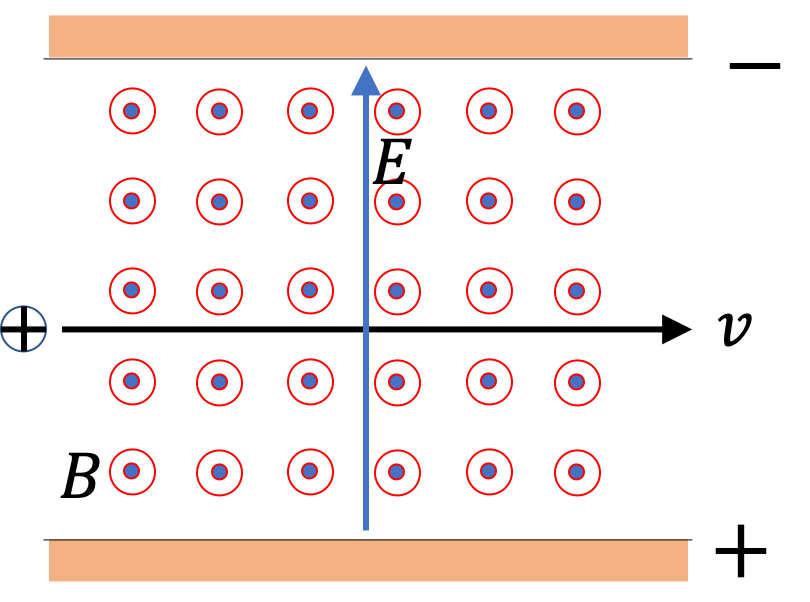A charged particle entering the region with its velocity perpendicular to both $\vec E$ and $\vec B$ will have oppositely directed electric and magnetic foeces acting on it. Balancing the two forces gives the condition for zero acceleration. Let us denote this particular speed to by $v_\text{selected}\text{.}$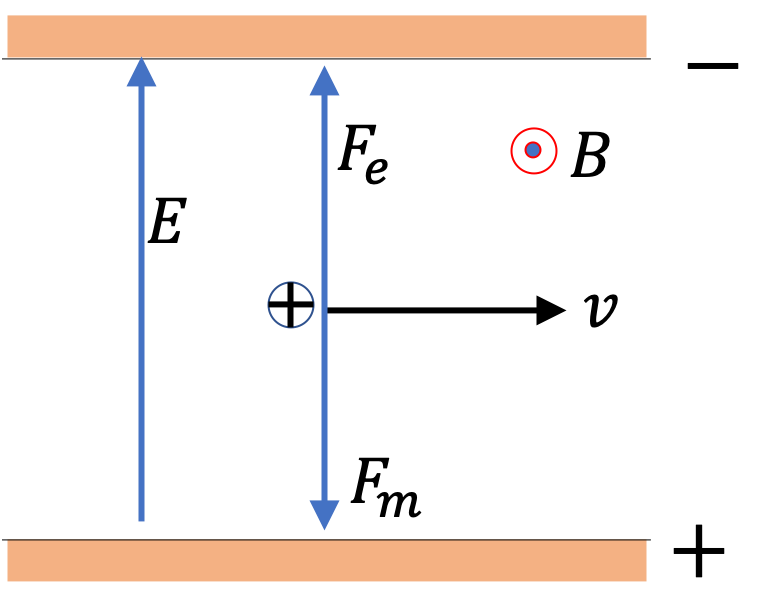\begin{equation*} qv_\text{selected} B = qE\Longrightarrow v_\text{selected} = \dfrac{E}{B}. \end{equation*}

If $v \gt v_\text{selected} \text{,}$ then magnetic force is greater and if $v \lt v_\text{selected} \text{,}$ then electric force is greater. In both cases, particle curves up or down. If we collect particles that pass through the region undeviated, i.e., in the straight path, we will selected particles with speed equal to $\dfrac{E}{B}\text{.}$ This is why a crossed-field region is called a velocity selector.

Suppose, for some experiment you need charged particles with speed $2.0\times 10^6\text{ m/s}\text{.}$ You have an apparatus that can generate a voltage of $3000\text{ V}\text{.}$ You apply this voltage across a parallel plate capacitor with distance $1.0\text{ cm}\text{.}$ What magnitude magnetic field do you need?

Hint

Use $E=V/d$ and $v=E/B\text{.}$

$0.15\text{ T}\text{.}$

Solution

From voltage across the parallel plate capacitor we get the electric field between the plates to be

\begin{equation*} E = \dfrac{V}{d} = \dfrac{3000\text{ V}}{1.0\times 10^{-2}\text{ m}} = 3\times 10^5\text{ V/m}. \end{equation*}

Now, we use the velocity-selector formula to get the needed $B\text{.}$

\begin{equation*} B = \dfrac{E}{v} = \dfrac{3\times 10^5}{2.0\times 10^{6}} = 0.15\text{ T}. \end{equation*}

An electron of speed $5 \times 10^6$ m/s moves in a circle of radius 3 cm when subjected to a magnetic field perpendicular to its velocity. (a) Find the magnetic field. (b) Evaluate the cyclotron frequency.

Hint

(a) Use $mv=QBR\text{.}$ (b) Use $f_c$ formula.

(a) Magnitude $9.5\times 10^{-4}\ \text{T}\text{,}$ (b) $\Omega = 1.7\times 10^8 \ \text{rad/s}$ or $f_c=2.66\times 10^{7}\text{ Hz}$ .

Solution 1 (a)

(a) Use the circular motion for a particle moving a magnetic field to obtain

\begin{align*} \dfrac{Q}{m} \amp = \dfrac{v}{RB}\ \ \Longrightarrow\ \ B = \dfrac{mv}{QR}\\ \amp = \dfrac{9.1\times 10^{-31}\:\text{kg}\times 5\times 10^{6}\:\text{m/s}}{1.6\times 10^{-19}\:\text{C}\times 0.03\:\text{m}} = 9.5\times 10^{-4}\:\text{T}. \end{align*}
Solution 2 (b)

(b) The cyclotron (angular) frequency is

\begin{align*} \Omega \amp = \dfrac{QB}{m}\\ \amp = \dfrac{1.6\times 10^{-19}\:\text{C} \times 9.5\times 10^{-4}\:\text{T}}{9.1\times 10^{-31}\:\text{kg}} = 1.67\times 10^{8}\:\text{rad/s}. \end{align*}

Therefore, cyclotron frequqncy is

\begin{equation*} f_c = \dfrac{\Omega}{2\pi} = 2.66\times 10^{7}\text{ Hz}. \end{equation*}

In a region between north and south poles of a strong magnet there is a uniform magnetic field of 3 T pointed up. An alpha particle of charge $+2\ e$ and mass equal to the sum of two protons and two neutrons enters the region from east with a speed of $4 \times 10^6$ m/s. It makes a circular arc of 90-degrees before exiting.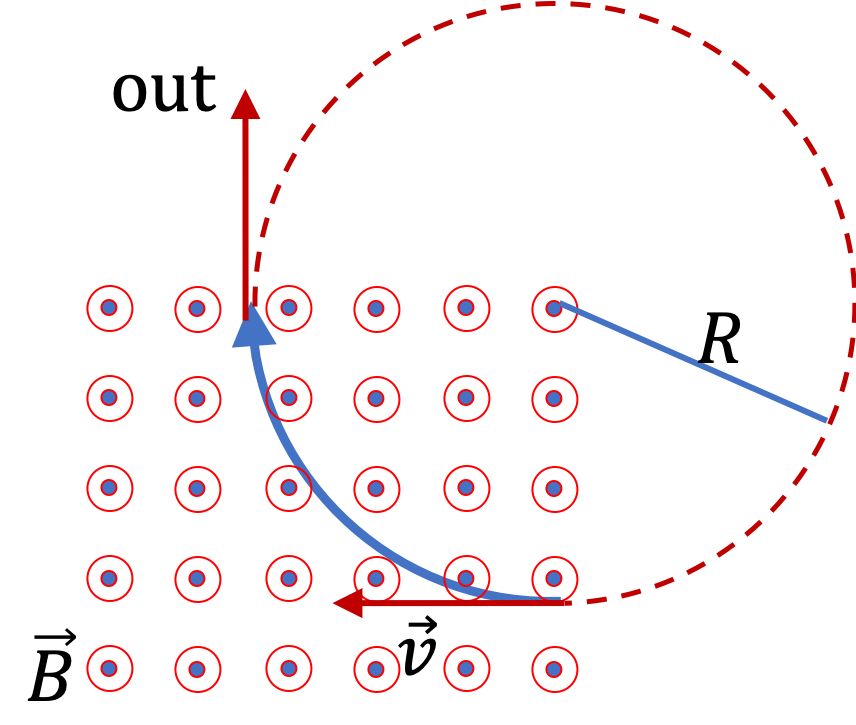(a) What is the radius of the circular arc? (b) What is the value of the cyclotron frequency?

Hint

Use circular motion equations.

(a) 2.78 cm, (b) $\Omega = 1.44\times 10^8\ \text{rad/s}$ or $f_c = 2.29\times 10^7\text{ Hz}.\text{.}$

Solution 1 (a)

(a) The radius of the circular arc will be

\begin{align*} R \amp = \dfrac{mv}{QB} = \dfrac{4\times 1.67\times 10^{-27}\:\text{kg}\times 4\times 10^{6}\:\text{m/s}}{2\times 1.6\times 10^{-19}\:\text{C}\times 3\:\text{T}} = 2.78\:\text{cm}. \end{align*}
Solution 2 (b)

(b) The cyclotron (angular) frequency will be

\begin{align*} \Omega \amp = \dfrac{QB}{m}= \dfrac{2\times 1.6\times 10^{-19}\:\text{C} \times 3\:\text{T}}{4\times 1.67\times 10^{-27}\:\text{kg}} = 1.44\times 10^{8}\:\text{rad/s}. \end{align*}

In units of $Hz$ this will be

\begin{equation*} f_c = \frac{\omega}{2\pi} = 2.29\times 10^7\text{ Hz}. \end{equation*}

A proton enters a uniform magnetic field region with a velocity of $6 \times 10^5 \text{ m/s}$ at an angle of $80^{\circ}$ with the direction of the magnetic field. Find the pitch of the helical path if magnetic field has a magnitude of $2\text{ T}\text{.}$

Hint

Use helical path equations.

$3.4\text{ mm}\text{.}$

Solution

To find the pitch we need the cyclotron frequency $\Omega$ and $z$-component of initial velocity, where $z$ is the axis of the helix.

\begin{equation*} \Omega = \dfrac{QB}{m} = \dfrac{1.60\times 10^{-19}\text{ C}\times 2\text{ T} }{ 1.67 \times 10^{-27}\text{ kg} } = 1.92\times 10^{8}\text{ rad/s}. \end{equation*}
The component of velocity along axis is
\begin{equation*} v_z = v\cos\,\theta = 6 \times 10^5\text{ m/s}\times \cos\, 80^{\circ} = 1.04\times 10^{5}\text{ m/s}. \end{equation*}
Therefore, pitch along axis is
\begin{equation*} \lambda = \dfrac{2\pi v_z}{\Omega} = 0.0034\text{ m} = 3.4\text{ mm}. \end{equation*}

A particle's path is bent when it passes through a region of non-zero magnetic field although its speed remains unchanged. This is very useful for beam steering in particle accelerators.

Consider an electron of speed $4 \times 10^6\text{ m/s}$ entering a region of uniform magnetic field $0.5\times 10^{-4}\text{ T}$ over a $10\text{-cm}$ wide region. Magnetic field is perpendicular to the velocity of the particle as shown in Figure 38.6.10. By how much angle will the path of the electron be bent?

Hint

The particle goes in and comes out tangents to a circle.

$12.7^\circ\text{.}$

Solution

The particle will move at a constant speed since magnetic force on a charged particle affects only the direction of the velocity and not the magnitude. From the circular motion we find that the radius of the circular arc will be given by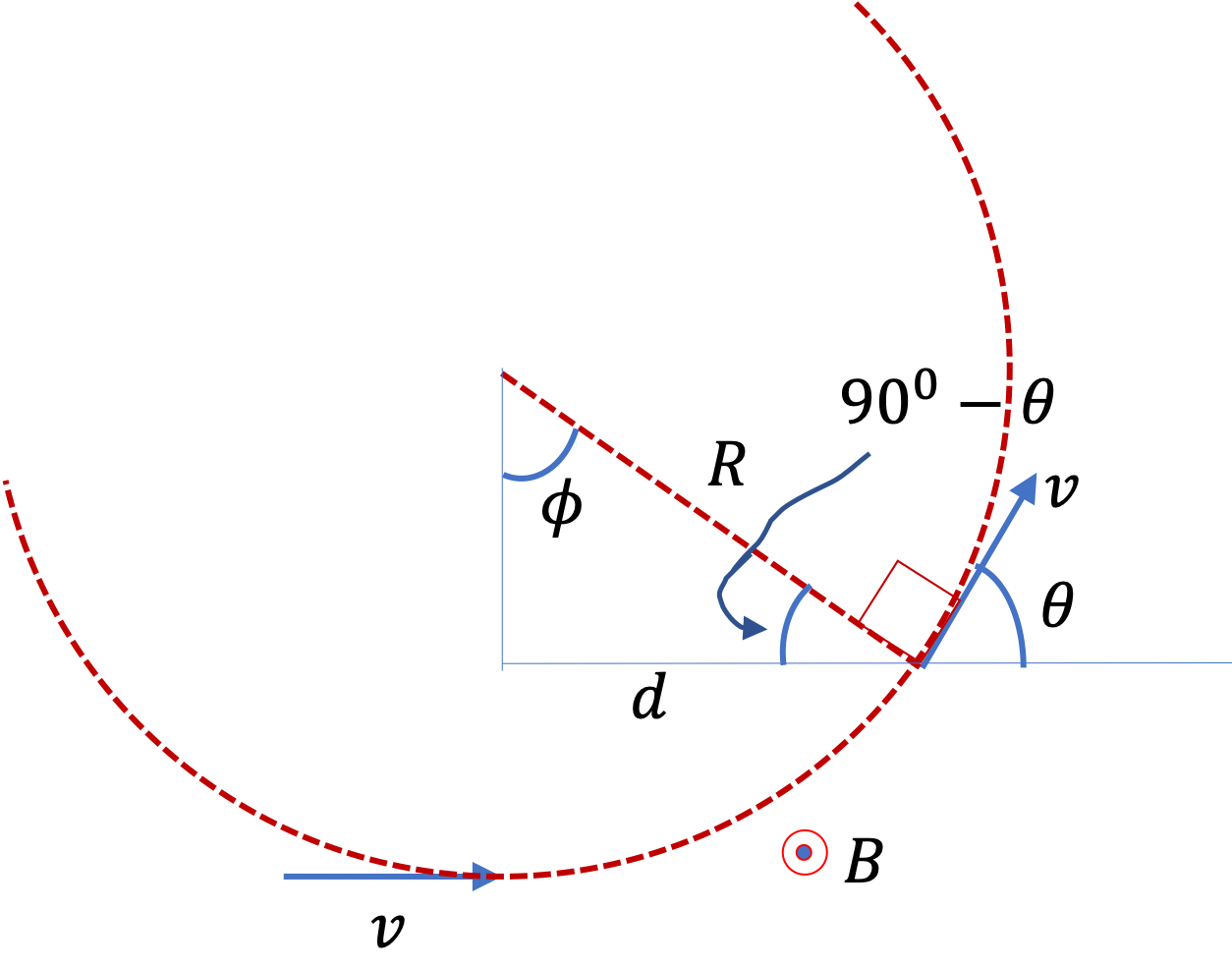\begin{align*} R \amp = \dfrac{mv}{|q|B}= \dfrac{9.1\times 10^{-31}\text{ kg} \times 4\times 10^{6}\text{ m/s}}{1.6\times 10^{-19}\text{ C}\times 0.5 \times 10^{-4}\text{ T}} = 0.455 \:\textrm{m}. \end{align*}

The angle of deviation $\theta$ can be obtained from the fact that particle's velocity is tangent to the circle of motion. Hence, from the figure it is seen that $\theta = \phi$ in the right-angled triangle. You can show this by making use of the fact that the tangent to a circle is normal to the line from the center. Therefore, the exit angle will be

\begin{align*} \theta \amp = \phi = \sin^{-1} \left( \dfrac{d}{R}\right) = \sin^{-1} \left( \dfrac{10\:\textrm{cm}}{45.5\:\textrm{cm}}\right) = 12.7^{\circ}. \end{align*}

A cyclotron is a device for accelerating charged particles. It was one of the earliest particle accelerators and it is still used today as a first stage in a multistage particle accelerator. Cyclotron uses electric field to boost the speed and magnetic field to bend the trajectory of the particle and bring it in a region where there is electric field to act on it again and again.

To accomplish this task a cyclotron used two D-shaped hollow metals with open flat side as shown in Figure 38.6.12. They are connected to an AC source of voltage, $V = V_0 \cos (2 \pi f t)\text{,}$ which creates an oscillating electric field in the gap between the two D's. Particle travels with increasing speed in larger and larger radii circles, but the time is same for each half-cycle.

A magnetic field is created perpendicular to the plane of D's by magnetic poles below and above the D's. Particles are injected in the center and accelerated into one of the D's where magnetic field bends it back into the gap, at which time electric field also is in the opposite direction to the last time particle was in the gap.

Particle travels with increasing speed in larger and larger radii circles, but the time is same for each half-cycle. The frequency the cyclotron frequency. The maximum speed achieved depends on the radius of the largest orbit. Let $Q$ and $m$ be the charge and mass of the particle, and $B_0$ be the uniform magnetic field.

Matching the cyclotron frequency to the frequency $f$ of the voltage oscillations required for proper operations of the cyclotron.

\begin{equation*} f = f_c = \dfrac{\Omega}{2\pi}= \dfrac{1}{2\pi}\:\dfrac{QB_0}{m}. \end{equation*}

Figure 38.6.14 shows an arrangement for measuring mass of ions by an instrument called mass spectrometer.

In a mass spectrometer, an ion created in a chamber is accelerated to a high speed by a accelerating voltage $V_\text{acc}\text{.}$ The ions of speed $v$ bend in a circular arc in a constant magnetic field $B_0\text{.}$ From the radius $R$ of the trajectory, $v\text{,}$ $q\text{,}$ and $B_0\text{,}$ we can find the mass of the ion.

(a) In Figure 38.6.14, the ion moves in a semi-circular path striking a photographic plate at a distance $x$ from the entry point. Derive a formula for mass $m$ in terms of $B_0\text{,}$ $q\text{,}$ $V_{\text{acc}}$ and $x\text{.}$

(b) In an experiment, we desire to have a separation of $\text{C}_2\text{H}_6^{+}$ and $\text{C}_2\text{H}_5\text{D}^{+}$ by $1.0\text{ mm}$ on the screen. We have $V_\text{acc}=550\text{ V}$ and we need to decide on the magnetic field we will need. Find the required magnetic field. Use masses of the ions to equal $30\,m_p$ and $31\,m_p$ .

Hint

(a) (1) Conservation of energy for the acceleration part, and (b) Circular motion for magnetic field par. (b) Use the answer of (a).

(a) $m = \left( \dfrac{qB_0^2}{8 V_{\textrm{acc}} }\right)\: x^2\text{,}$ (b) $0.0194\text{ T}\text{.}$

Solution 1 (a)

(a) In the acceleration part we apply conservation of energy to find the speed upon acceleration by electric field in the accelerator.

\begin{equation} \dfrac{1}{2}\,m v^2 = qV_{\text{acc}}\Longrightarrow v = \sqrt{2qV_{\text{acc}}/m}.\label{eq-mass-spec-acceleration}\tag{38.6.7} \end{equation}

In the region of the uniform magnetic field, the motion is uniform circular motion of radius $R\text{.}$

\begin{equation} R = \dfrac{mv}{qB_0}.\label{eq-mass-spec-bending-path}\tag{38.6.8} \end{equation}

Using $v$ from Eq. (38.6.7), we get

\begin{equation*} R = \dfrac{m}{qB_0}\times \sqrt{2qV_{\text{acc}}/m}. \end{equation*}

Solving for $m\text{,}$

\begin{equation*} m = \left( \dfrac{qB_0^2}{2 V_{\textrm{acc}} }\right)\: R^2. \end{equation*}

Since diameter $x = 2R\text{,}$ we can express this in $x$

\begin{equation*} m = \left( \dfrac{qB_0^2}{8 V_{\textrm{acc}} }\right)\: x^2. \end{equation*}
Solution 2 (b)

(b) From answer in (a) we have

\begin{equation*} x = 2R = \dfrac{1}{B_0}\times \sqrt{8mV_{\text{acc}}/q}. \end{equation*}

Therefore, for the two ions we will get

\begin{align*} x_2 - x_1 \amp = \dfrac{1}{B_0}\times \sqrt{8m_2V_{\text{acc}}/q} - \dfrac{2}{B_0}\times \sqrt{8m_1V_{\text{acc}}/q} \\ \amp = \dfrac{1}{B_0}\times \sqrt{8V_{\text{acc}}/q} \left(\sqrt{m_2} - \sqrt{m_1} \right). \end{align*}

Solving for $B_0$ gives

\begin{equation*} B_0 = \dfrac{\sqrt{8V_{\text{acc}}/q}}{x_2-x_1} \left(\sqrt{m_2} - \sqrt{m_1} \right). \end{equation*}

We now put in the numbers, converting all units in the SI. Using masses $m_1=30m_p$ and $m_2=31m_p$ and $m_p = 1.67\times 10^{-27}\text{ kg}\text{,}$ to get

\begin{align*} B_0 \amp = \dfrac{ \sqrt{8 \times 550/1.6\times 10^{-19}} }{0.001}\times \\ \amp \ \ \ \ \ \ \ \ \left(\sqrt{31\times 1.67 \times 10^{-27} } - \sqrt{30\times 1.67 \times 10^{-27} } \right) \\ \amp = 0.0194\text{ T}. \end{align*}